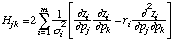# Manual Page for Command >>> HESSIAN

Syntax
>>> HESSIAN

Parent Command
>> ERROR
>> JACOBIAN

Subcommand
-

Description
This command computes a finite difference Hessian matrix H for the error analysis following optimization. The elements of H are given by:The evaluation of H by means of finite differences requires 2n+n*(n-1)/2 additional TOUGH2 simulations, where n is the number of parameters. By default, the Hessian matrix, which is the inverse of the parameter covariance matrix, is approximated bybased on the linearity assumption, i.e., the second derivative term is ignored. Evaluating the finite difference Hessian, which takes into account the non-linearities, provides a means by which to check the linearity assumption (for another approach see command >>> LINEARITY). This may lead to a more accurate calculation of the covariance matrix of the estimated parameters. However, inclusion of the second-derivative term may yield a Hessian matrix that is not positive definite due to the presence of outliers, strong non-linearities, or the fact that the minimum has not been detected accurately, i.e., when the positive and negative residuals r do not cancel each other. In this case, iTOUGH2 automatically proceeds with the linearized Hessian which is positive definite by definition.

Example
> COMPUTATION
>> ERROR analysis should be based on
>>> finite difference approximation of the HESSIAN matrix
<<<